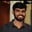Related Tags

matrix
transpose
c
communitycreator

# How to transpose a rectangular matrix in CBuchiredddypalli Koushik

### Problem statement

We need to read the elements of a matrix using the standard input (scanf) and transpose the matrix to get the result. In the solution code we’ll read the input of rows and columns of the matrix from the standard input. The result should be stored in the new matrix transpose.

### Sample problem

Let’s first take a 2x3 matrix and transpose it.

Original Matrix:

$\begin{bmatrix} 1 & 2 & 3 \\ 4 & 5 & 6 \end{bmatrix} \quad$

Transpose Matrix: The resultant matrix will look like this:

$\begin{bmatrix} 1 & 4 \\ 2 & 5 \\ 3 & 6 \end{bmatrix} \quad$

### Discussion

In the transpose matrix, the rows will be exchanged with columns and vice versa.

### Algorithm

Generally, the transpose of the matrix is denoted by T. The transpose of the matrix means that we need to change its rows into columns or columns into rows. When reading the data, we need to use loops. We also need loops to access the transpose of the matrix. After transposing the elements, we store the data in the resultant matrix.

### Pseudocode of the algorithm

for(i=0 ; i<rows ; i++) {
for(j=0 ; j<cols  ; j++) {
trans[j][i] =a[i][j];
}
}


### Notations

• The rows represent the size of the rows.
• The cols represent the size of the columns.
• The a[rows][cols] represents the original matrix.
• The trans[cols][rows] represents the transpose of the matrix or the resultant matrix.
1 of 2

### Input format for the code

To run the code below, give the input value for rows and cols followed by element values of the matrix.

After giving all the inputs, we get the resultant matrix which is the transpose of the input matrix.

#include <stdio.h>
#include <string.h>
#include <math.h>
#include <stdlib.h>
int  main()
{
int rows,cols; // Declaring the variables
scanf("%d %d",&rows,&cols);
if(rows <= 0 || cols <=0) {
printf("Values for rows and coulmns must be positive and greater than zero.\n");
exit(0);
}
int a[rows][cols] ; // Declaring the matrix
int trans[cols][rows] ;// Declaring the transpose matrix
// Reading of the original matrix
int value_count = 0 ;
for(int i=0;i<rows;i++)
{
for(int j=0;j<cols;j++)
{
scanf("%d",&a[i][j]);
value_count++;
}
}
// Transposing the matrix
for(int i=0;i<rows;i++)
{
for(int j=0;j<cols;j++)
{
trans[j][i]=a[i][j];
}
}
// Printing the transpose matrix
int i,j;
for(i=0;i<cols;i++)
{
for( j=0;j<rows;j++)
{
printf("%d ",trans[i][j]);
}
printf("\n");
}
return 0;
}

Enter the input below

Transpose of a rectangular matrix

### Explanation

• In the above code first we will be reading the size for the rows and columns.

• After reading the size then we will be reading a matrix from the user end.

• Then we will interchange the rows with columns or columns with rows. As soon as we interchange the rows and columns we print the matrix.

RELATED TAGS

matrix
transpose
c
communitycreator

CONTRIBUTORBuchiredddypalli Koushik
RELATED COURSES

View all Courses

Keep Exploring

Learn in-demand tech skills in half the time